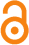Mathematical Analysis of the Impact of Vaccination in the Spread of Diseases in a Large Susceptible Population

B. Barnes , A. Y. Aidoo , K. F. Darkwah , E. Osei-Frimpong 

 School of Agricultural and Bio-resource Engineering, Anglican University College of Technology, Nkoranza-Ghana

 Department of Mathematics and Computer Science, Eastern Connecticut State University, U. S. A.

 Department of Mathematics, Kwame Nkrumah University of Science and Technology, Kumasi-Ghana

Abstract

In this paper, we have provided the threshold condition for an outbreak of the disease that is characterized by equal birth/death rate and proportion of vaccinated population subjects. Our result mimics the essential features of the disease and provides valuable insights into epidemiological process. Our work unvails no periodic nature of acquisition of measles, mumps and rubella diseases. However, we have used sensitivity analysis to show that administering of vaccine on every unit of population subjects does not imply permanent immunity. The system is characterized by two equilibrium states which become local asymptotically stable when certain biological parameters are controlled. The disease equilibrium state is global asymptotically stable if the proportion of susceptible is greater or equal to proportion of infectives. Also, it is stable (global) when the sum of birth/death rate and recovery rate is greater or equal to the product of transmission rate and susceptible proportion.

Keywords: non-dimensionalization, threshold theorem, equilibrium states, global stability, limit cycle solution, sensitivity analysis, constant vaccination strategy.

Citation: Barnes, B., Aidoo, A. Y., Darkwah, K. F., & Osei-Frimpong, E. (2013). Mathematical Analysis of the Impact of Vaccination in the Spread of Diseases in a Large Susceptible Population. Open Science Repository Mathematics, Online(open-access), e23050423. doi:10.7392/openaccess.23050423

Published: September 25, 2013

Contact: research@open-science-repository.com

Full text

Cite this paper

APA

Barnes, B., Aidoo, A. Y., Darkwah, K. F., & Osei-Frimpong, E. (2013). Mathematical Analysis of the Impact of Vaccination in the Spread of Diseases in a Large Susceptible Population. Open Science Repository Mathematics, Online(open-access), e23050423. doi:10.7392/openaccess.23050423

MLA

Barnes, B. et al. “Mathematical Analysis of the Impact of Vaccination in the Spread of Diseases in a Large Susceptible Population.” Open Science Repository Mathematics Online.open-access (2013): e23050423.

Chicago

Barnes, B., A. Y. Aidoo, K. F. Darkwah, and E. Osei-Frimpong. “Mathematical Analysis of the Impact of Vaccination in the Spread of Diseases in a Large Susceptible Population.” Open Science Repository Mathematics Online, no. open-access (September 25, 2013): e23050423. doi:10.7392/openaccess.23050423.

Harvard

Barnes, B. et al., 2013. Mathematical Analysis of the Impact of Vaccination in the Spread of Diseases in a Large Susceptible Population. Open Science Repository Mathematics, Online(open-access), p.e23050423.

Science

1. B. Barnes, A. Y. Aidoo, K. F. Darkwah, E. Osei-Frimpong, Mathematical Analysis of the Impact of Vaccination in the Spread of Diseases in a Large Susceptible Population, Open Science Repository Mathematics Online, e23050423 (2013).

Nature

1. Barnes, B., Aidoo, A. Y., Darkwah, K. F. & Osei-Frimpong, E. Mathematical Analysis of the Impact of Vaccination in the Spread of Diseases in a Large Susceptible Population. Open Science Repository Mathematics Online, e23050423 (2013).

doi

Research registered in the DOI resolution system as: 10.7392/openaccess.23050423.